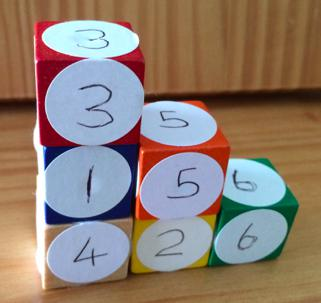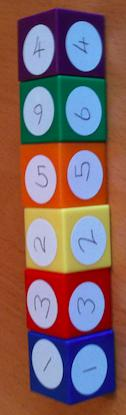#### You may also like### Pebbles

Place four pebbles on the sand in the form of a square. Keep adding as few pebbles as necessary to double the area. How many extra pebbles are added each time?### Bracelets

Investigate the different shaped bracelets you could make from 18 different spherical beads. How do they compare if you use 24 beads?### Sweets in a Box

How many different shaped boxes can you design for 36 sweets in one layer? Can you arrange the sweets so that no sweets of the same colour are next to each other in any direction?

# Six Numbered Cubes

##### Age 7 to 11Challenge Level

The aim of this challenge is to find the total of all the visible numbers on the cubes.

We are using six cubes.
Each cube has six faces of the same number.

.The "wall" has to be only one cube thick.
The one on the left is built correctly by being a wall only one cube thick.
The one on the right is NOT allowed as it is two bricks thick in parts.
The cubes sit neatly - square face against square face.
The total on the left one is 70.

CHALLENGE 1

Start by making a staircase shape.  An example is shown below:a/ What is the highest total you can make by using this staircase shape?

b/ What is the lowest total you can make by using this staircase shape?

c/ Explain in writing how you calculated the totals for a & b above, making sure you give reasons for your method/s.

d/ Now make a total of 75 using a staircase shape.

CHALLENGE 2

Using any shape of single cube thickness, what is the lowest total you can make?
How can you be sure this is the lowest total whatever the shape?
Can the lowest total be found in more than one way? Justify your answer.

CHALLENGE 3

Using any shape of single cube thickness, what is the highest total you can make?
How can you be sure this is the highest total whatever the shape?
Can the highest total be found in more than one way? Justify your answer.

CHALLENGE 4

Prove the following by logical reasoning, rather than by calculating the answers:

If the cubes are arranged in a single vertical tower (like this)then whatever the order of cubes you cannot produce a total of 80.

This problem featured in a round of the Young Mathematicians' Award 2014.